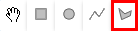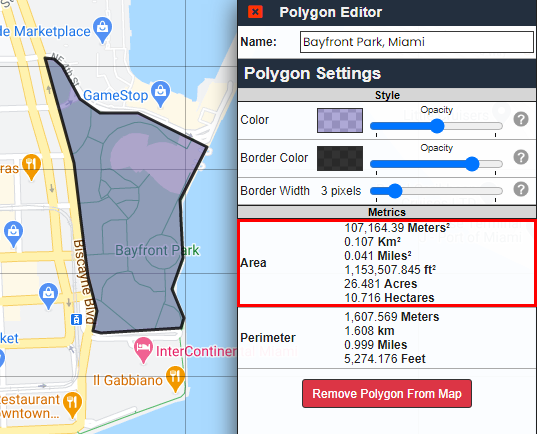# Calculating Land / Surface Area

Calculating land / surface area is easy with Map Maker. Area is automatically calculated when you create or draw an "enclosed" shape on the map, i.e. a polygon, rectangle or circle.

To view the calculated area, click on the object or the layer that represents the object. The calculated area will be displayed under "Metrics" in the slide-out Editor section.

The area is calculated in a number of units, e.g. square meters, square miles, acres, etc.

Below is an example for calculating the land area of a polygon shape on the map.

## Example: Calculating land area of a polygon

Step 1: Click the polygon drawing tool to enable the polygon drawing functionStep 2: Draw the desired polygon on the map by clicking the points on the map that outline the area you would like to measure.

Be sure that you close the polygon, i.e. the final click that outlines the polygon should be the same as the first/origin point.

Step 3: Once the shape is drawn, click on the polygon. This will launch the "Polygon Editor" to the right.

In the Polygon Editor, there will be an "Area" row under the "Metrics" section. This will contain the calculated area: